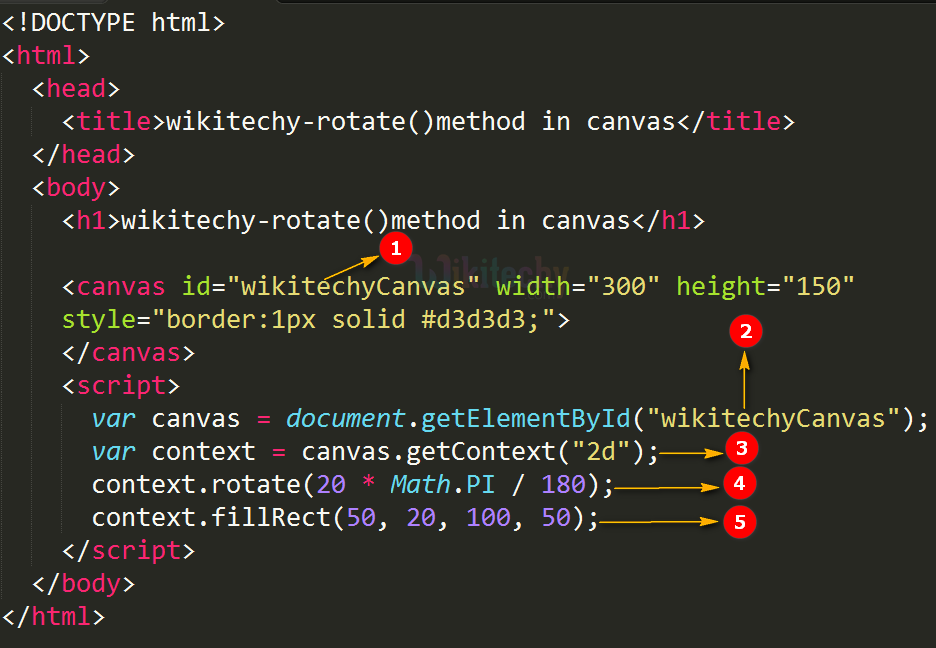# html tutorial - rotate() Method in HTML5 Canvas - html5 - html code - html formLearn html - html tutorial - Rotate method in html5 canvas - html examples - html programs

• The rotate() is the method of HTML CANVAS
• The rotate() method is used to rotate the current diagram.

## Parameter values for rotate() Method in HTML5 Canvas:

Parameter Description
angle The rotation angle, in radians.

## Code Explanation for rotate() Method in HTML5 Canvas:1. ”wikitechyCanvas” is used to define the id attribute value for canvas element.
2. The getElementById(); method is used to get the element that has the id attributes value as “wikitechyCanvas”.
3. canvas.getContext() method returns an object that provides methods and properties for drawing two dimension figure on the canvas
4. The beginPath() method is used to begins the path for a line.
5. mak.lineWidth is used to set a line width as 10.
6. The context.rotate() method is used to rotate a diagram at (20 * Math.PI / 180) radiant.
7. The context.fillRect() method is used to draw a "filled" rectangle. (50, 20, 100, 50);

## Output for rotate() Method in HTML5 Canvas:1. <canvas> tag is used to draw the rectangle with blue color border.
2. The output shows that a 20-degree rotated rectangle.

## Browser Support for rotate() Method in HTML5 Canvas:Yes 9.0 Yes Yes Yes